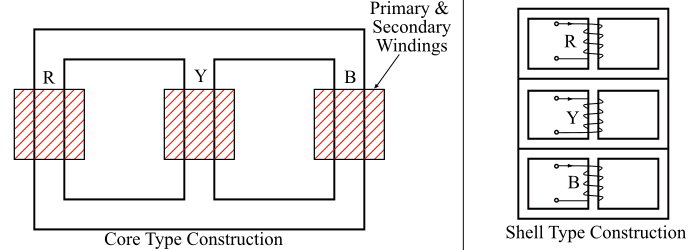# Working Principle of a Three-Phase Transformer

A three phase transformer is required to step-up or step-down the three phase voltages in a power system. The 3-phase transformers can be constructed in one of the following ways −

• Three separate 1-phase transformers can be connected for the 3-phase operation. Such an arrangement is known as 3-phase bank of transformers.

• A single three phase transformer can be constructed in which the cores and windings for all the three phases are combined in a single structure.

## Construction of a Three Phase Transformer

The figure shows the core type and the shell type construction of the three-phase transformer. The 3-phase transformer consists of a magnetic core and the three phase windings which are connected in star or delta according to the requirement.### Core Type Construction

In the core type construction of 3-phase transformer, the magnetic core is built up of laminated steel sheets. The core consists of three limbs in the same plane and each limb carries both lowvoltage (lv) winding and high-voltage (hv) winding.

As it is easier to insulate the lv-windings from the core than the hv-windings, the low-voltage windings are placed next to the core with required insulation between the core and the low voltage windings. The high voltage windings are placed over the low voltage windings with required insulation between them.

### Shell Type Construction

The 3-phase shell type transformer is constructed by stacking three 1-phase shell type transformers. The direction of the windings of the central unit (unit-Y) is made opposite to that of units R and B.

## Working Principle of Three-Phase Transformer

The primary winding of the three phase transformer is energized from a three phase supply. Then, the flux is produced in the core by the primary currents in the three windings. The core has three limbs, where any two limbs act as return path for the flux in the third limb. The flux produced by the primary windings induces emf in the secondary windings depending on the transformation ratio of the 3-phase transformer.

### 3-Phase Transformer Transformation ratio

In case of a three phase transformer, the transformation ratio is defined as the ratio of the secondary phase voltage to the primary phase voltage and is denoted by K.

$$\mathrm{Transformation\:ratio,\:𝐾 =\frac{Secondary\:phase\:voltage\:(𝑉_{2𝑝ℎ})}{Primary\: phase\:voltage\:(𝑉_{1𝑝ℎ})}}$$

## Numerical Example

A 3-phase, 50 Hz transformer has a delta connected primary winding and star connected secondary winding, the line voltages are 11000 V and 440 V respectively. A star connected balanced load of p.f. 0.8 lagging is connected to the secondary winding. The primary side line current is 10 A. Determine the current in each phase of the primary winding and in each line of secondary winding. Also, calculate the output power of the transformer in kW.

Solution

$$\mathrm{Primary\:phase\:voltage,\:𝑉_{1𝑝ℎ} = 11000\:V}$$

$$\mathrm{Secondary\:phase\:voltage,\:𝑉_{2𝑝ℎ} =\frac{440}{√3}= 254\:V}$$

$$\mathrm{∴\:Transformation\:ratio,\:𝐾=\frac{𝑉_{2𝑝ℎ}}{𝑉_{1𝑝ℎ}}=\frac{254}{11000}= 0.0231}$$

Therefore, the current in each phase of the primary side is

$$\mathrm{Primary\:phase\:current,\:𝐼_{1𝑝ℎ} =\frac{10}{√3}= 5.77\:A}$$

$$\mathrm{∴\:Secondary\:phase\:current, 𝐼_{2𝑝ℎ}=\frac{𝐼_{1𝑝ℎ}}{𝐾}=\frac{5.77}{0.0231}= 249.78 \:A}$$

Thus, the current in each line on secondary side is

$$\mathrm{Secondary\:line\:current,\:𝐼_{2𝐿} = 𝐼_{2𝑝ℎ} = 249.78\:A}$$

The output power the transformer is given by,

$$\mathrm{𝑃_{𝑜} = √3 𝑉_{𝐿} 𝐼_{𝐿}\:cos\:φ = √3 × 440 × 249.78 × 0.8 = 152.281\:kW}$$

Updated on: 14-Aug-2021

9K+ Views